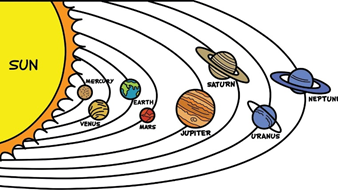# NCERT Class VIII: Science-Exercise Solutions

### Chapter 17–Stars and the Solar System-Exercise Solutions

NCERT Book Page Number-234
Question 1:
Q.1. Which of the following is NOT a member of the solar system?
(a) An asteroid
(b) A satellite
(c) A constellation
(d) A comet
(c) Constellation
A constellation is not a member of the solar system. Constellations are groups of stars that form recognisable shapes.

Q.2. Which of the following is NOT a planet of the sun?
(a) Sirius
(b) Mercury
(c) Saturn
(d) Earth
(a) Sirius
Sirius is a star and not a planet of the sun.
Q.3. Phases of the moon occur because
(a) we can see only that part of the moon which reflects light towards us.
(b) our distance from the moon keeps changing.
(c) the shadow of the Earth covers only a part of the moon’s surface.
(d) the thickness of the moon’s atmosphere is not constant.
(a) Phases of the moon occur because we can see only that part of the moon which reflects light towards us.
Moon does not produce its own light. We are able to see the moon because the sunlight falling on it gets reflected towards us. Thus, we see only that part of the moon from which the light of the sun gets reflected towards us.
Q.4. Fill in the blanks:
(a) The planet which is farthest from the sun is _________.
(b) The planet which appears reddish in colour is _________.
(c) A group of stars that appear to form a pattern in the sky is known as a __ _________.
(d) A celestial body that revolves around a planet is known as a _________.
(e) Shooting stars are actually not _________.
(f) Asteroids are found between the orbits of ________ __ and _________.
(a) The planet which is farthest from the sun is __Neptune__.
(b) The planet which appears reddish in colour is __Mars__.
(c) A group of stars that appear to form a pattern in the sky is known as a __constellation__.
(d) A celestial body that revolves around a planet is known as a satellite_.
(e) Shooting stars are actually not stars __.
(Shooting stars are not stars, they are meteors)
(f) Asteroids are found between the orbits of __Mars __ and __Jupiter.
(Asteroids occupy a large gap between the orbits of Mars and Jupiter)
Q.5. Mark the following statement as true (T) or false (F).
(a) Pole star is a member of the solar system. ( )
(b) Mercury is the smallest planet of the solar system. ( )
(c) Uranus is the farthest planet in the solar system. ( )
(d) INSAT is an artificial satellite. ( )
(e) There are nine planets in the solar system. ( )
(f) Constellation Orion can be seen only with a telescope. ( )
(a) False
Stars are not a member of the solar system. The sun and the celestial bodies revolving around it form the solar system.
(b) True
Mercury is the smallest planet of the solar system.
(c) False
Neptune is the farthest planet in the solar system.
(d) True
INSAT is an artificial satellite.
(e) False
There are eight planets in the solar system.
They are Mercury, Venus, Earth, Mars, Jupiter, Saturn, Uranus and Neptune.
(f) False
Constellation Orion can be seen during winters around late evenings. It is one of the most magnificent constellations in the sky, visible to the naked eyes.
NCERT Book Page Number-235

Q.6. Match items in column A with one or more items in column B.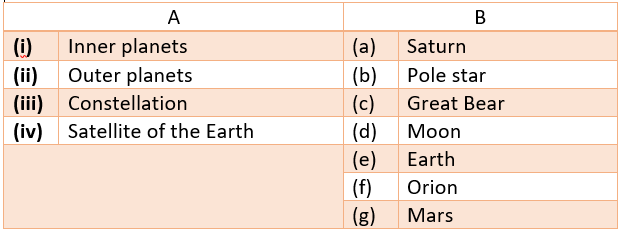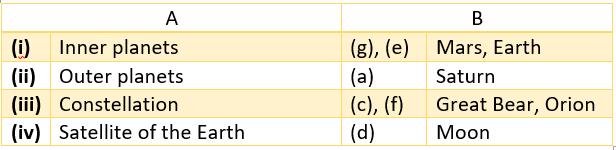Q.7. In which part of the sky can you find Venus if it is visible as an evening star?
Answer: Venus appears in the western sky after sunset and is called the evening star.
Q.8. Name the largest planet of the solar system.
Answer: The largest planet of the solar system is Jupiter.
Q.9. What is a constellation? Name any two constellations.
Answer: A constellation is a group of stars that form a recognisable pattern in the sky.
The two well-known constellations are Ursa Major and Orion.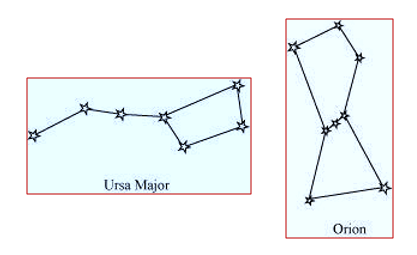Q.10. Draw sketches to show the relative position of prominent stars in (a) Ursa Major and (b) Orion
Answer: (a) Ursa Major appears like a big dipper. There are three bright stars in the handle and four stars in the bowl of the dipper (as shown in the given figure).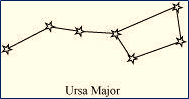(b) Orion appears like a hunter. Three bright stars appear in the belt, while five bright stars are arranged in the form of a quadrilateral (as shown in the given figure).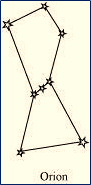Q.11. Name two objects other than planets which are members of the solar system.
(i) Asteroids
A collection of a large number of small objects, gases and dust are revolving around the sun. They occupy a large gap between the orbits of Mars and Jupiter. However, these are not planets. These celestial objects are known as asteroids.
(ii) Meteors
Meteors are small celestial objects that are seen as bright streaks of light in the sky. They brunt out on entering the Earth’s atmosphere because of the heat produced by friction. This results in bright streaks in the sky. They are not planets.
NCERT Book Page Number-237
Q.12. Explain how you can locate the Pole Star with the help of Ursa Major.
Answer: In order to locate the Pole star in the sky, first of all Ursa Major or Big Dipper constellation must be located. The bowl of the Big Dipper consists of four bright stars (as shown in the given figure).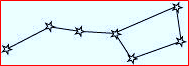Consider two stars at the end of this bowl. Now, draw an imaginary straight line towards the Northern direction connecting these two stars (as shown in the given figure).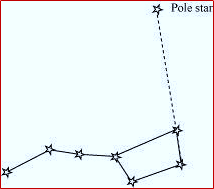This imaginary line meets a star called the Pole Star. The length of the imaginary line from the bowl is about five times the distance between the two stars of the bowl.
Q.13. Do all the stars in the sky move? Explain.
Answer: No. The Earth rotates from West to East on its axis. Hence, all stars in the sky (except the Pole star) appear to move from East to West. With reference to the Earth, the Pole star does not appear to move in the sky because it is located above the axis of rotation of the Earth in the north direction. It appears to remain stationary at a point in the sky.
Q.14. Why is the distance between stars expressed in light years? What do you understand by the statement that a star is eight light years away from the Earth?
Answer: The distance of the stars from the Earth and the distance between the stars are very large. It is inconvenient to express these distances in kilometer (km). Thus, these large distances are expressed in light years. One light year is the distance travelled by light in one year. One light year is equal to 9.46 × $10^{12}$ km.
A star is located eight light years away from the Earth. This means that the distance between the star and the Earth is equivalent to the distance travelled by light in eight years, i.e., a star is located 8 × (9.46 × $10^{12}$) = 7.6 × $10^{13}$ km away from the Earth.

Q.15. The radius of Jupiter is 11 times the radius of the Earth. Calculate the ratio of the volumes of Jupiter and the Earth. How many Earths can Jupiter accommodate?
Answer: Earth and Jupiter can be considered as two spheres with radii R and R’ respectively. Given that the radius of Jupiter is 11 times the radius of the Earth.
Thus, R’ = 11 R
Volume of a sphere of radius r is given as = $\frac{4}{3}\pi r^{3}$
Volume of the Earth = $\frac{4}{3}\pi R^{3}$

And, volume of Jupiter = $\frac{4}{3}\pi R’^{3}$
= $\frac{4}{3}\pi (11R)^{3}$
= 1331$\frac{4}{3}\pi R^{3}$

The ratio of the volumes of Jupiter and Earth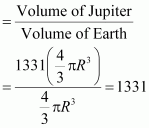Hence, this ratio suggests that Jupiter can accommodate 1331 number of Earths within it.

Q.16. Boojho made the following sketch (Fig. 17.29) of the solar system. Is the sketch correct? If not, correct it.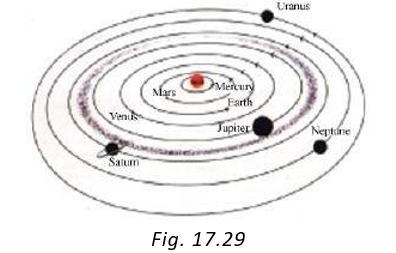Answer: Planets of the solar system in sequence of their distances from the sun are: Mercury, Venus, Earth, Mars, Jupiter, Saturn, Uranus, and Neptune. Therefore, Boojho’s sketch of the solar system is not correct because he has interchanged the positions of Mars and Venus and also the positions of Uranus and Neptune. Also, he has shown the Asteroid belt in the gap between the orbits of Jupiter and Saturn. This is not correct. The asteroid belt is located between the orbits of Mars and Jupiter. The correct sketch of the solar system is shown in the given figure.